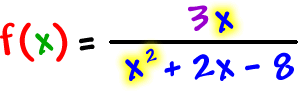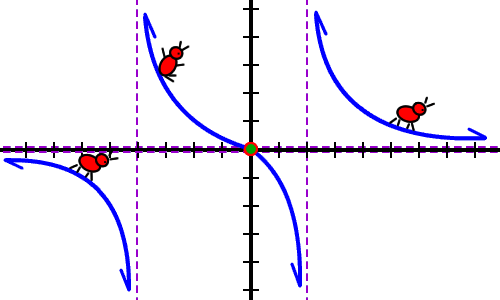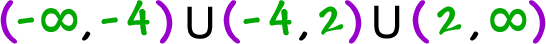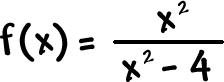It's one wild ride for Pierre!  Downhill sledding all the way!  But, if we said that f is decreasing everywhere, that wouldn't be quite right...  Because the graph doesn't exist at x = -4 and x = 2.

It's decreasing everywhere on its domain, which isGivenFind the intervals where f is decreasing.

Find the intervals where f is increasing.

 GivenFind the intervals where f is decreasing.

Find the intervals where f is increasing.